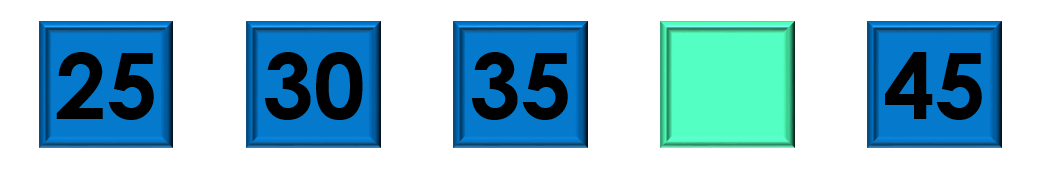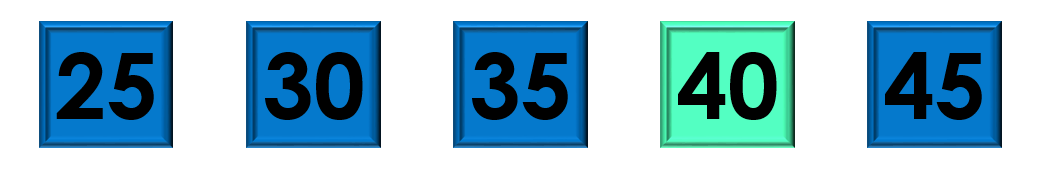1
visibility

Consider the given number pattern and identify the missing number.• A

43

• B

40

• C

45

• D

46

Solution:

Considering the given number pattern.Here, the numbers are increasing.
So, to find the missing number, we first find the rule.

Note that:
25 + 5 = 30
30 + 5 = 35
35 + 5 = 40
40 + 5 = 45

So, the rule is "add 5".Then, the missing number is 40.